Next: Learning Module Exercise Up: Analog and Digital Interfacing Previous: Mechanical Switches

This section discusses methods for driving resistive and capacitive loads from the MicroStamp11's output pins. Some care must be taken in interfacing such loads to an output pin in order to prevent damaging the MicroStamp11. To see how such damage might occur, let's consider consider the circuit shown below in figure 17.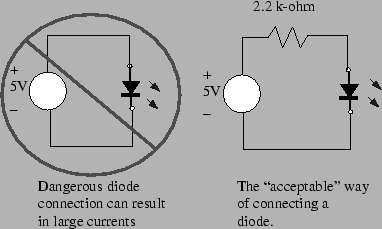The output pin of the MicroStamp11 can be modelled as an independent 5 volt voltage source when the pin's logical state is "high". So let's see what happens if we use the left hand circuit in figure 17. This circuit directly connects the output pin to ground through a light emitting diode (LED). Because of the diode's orientation, this diode is forward biased. A forward biased diode will drop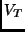volts (is the diode's threshold voltage and it lies somewhere between .7 to 1.6 volts depending on the type of semiconductor material used in the diode). But the forward biased device is, essentially, a short circuit which means its resistance is essentially zero. By Ohm's law, the current,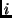, passing through the diode will be given by the equation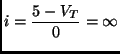The above equation means that the diode will try to pass an infinite current. In reality, of course, the MicroStamp11 cannot deliver this level of current for its peak output current is limited to about 10 mA. However, the device will try to meet the demand and in doing so it will probably damage the delicate circuitry within the IC. So, the left-hand circuit shown in figure 17 is highly undesirable. If we want to drive the LED we need to find some way to limit the current demand on the MicroStamp11.

A circuit that limits the current demand is shown in the right hand circuit in figure 17. Since the resistor,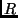, is in series with the diode, the current passing through the diode will be limited by the resistor. The current drawn by this circuit will be given by the formula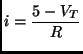whereis the current limiting resistor's resistance in ohms. As an example, let's assume that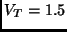volts and let's assume that we want to keep the current drawn out of the output pin below 1 mA. Then we require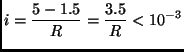Re-arranging terms, we find that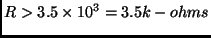In other words, to keep the current drawn out of the output pin below 1 mA, we need to put a 3.5 k-ohm resistor in series with the LED.

A similar problem will be encountered if we attempt to use the MicroStamp11 to drive a capacitor. The left hand circuit in figure 18 shows the output pin connected to ground through a capacitor,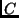. Now we know that the current,passing through a capacitor satisfies the relation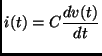whereis the capacitance (measured in farads) and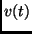is the voltage over the capacitor. So let's think about what happens in the circuit shown in figure 18. We letdenote the voltage at the output pin and we consider what happens when we "switch" the output pin's state from low to high at time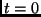. This means that the waveformis a step function of the form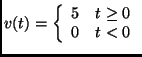According to the capacitor equation, we know that the current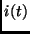is proportional to the derivative of the voltage. The derivativeis zero everywhere except at.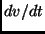is unbounded atand this means, therefore that when we switch the capacitor on, the MicroStamp11 will attempt to provide an infinite current through the capacitor for a short time instant. Such unbounded currents can obviously damage the MicroStamp11 and so we need to limit the current flow that can potentially flow through the cap in response to a step change in the applied output pin voltage.Once again,we need to use a resistor in series with the capacitor to limit the voltage through the capacitor. This "safer" circuit is shown in the right hand circuit in figure 18. The size of the current limiting resistor,is again determined by Ohm's law. Note that in response to an abrupt voltage change, the capacitor appears to be a short circuit. So the required resistance can be obtained from the equation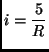Assuming, as before, that we want to limit the peak current drawn by the MicroStamp11 to less than 1 mA, the above equation implies that we need to use a resistance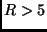kilo-ohms.

The preceding discussion has shown how we can use current-limiting resistor to restrict the current drawn out of the MicroStamp11 below a specified safe level. In general, however, we should realize that these current levels are very small and in certain cases, they may not be sufficiently large to adequately drive the load. As an example, we can consider the LED circuit shown in figure 17. The brightness of the LED is proportional to current flowing through it. To achieve an adequate brightness level, we may want to drive these LED's at 10 mA, rather than 1 mA.

We can drive our LED's with higher current, provided we introduce a buffer between the LED and the MicroStamp11. Two such circuits are shown below in figure 19. The left hand circuit uses an npn bipolar transistor as a current driver for the LED. A transistor is a 3-terminal semiconductor device that can act as an amplifier or an electronic switch. The three terminals are called the base, collector, and emitter. The labeling of these terminals is shown in figure 19. The circuit in figure 19 uses the transistor as a switch. The output pin is connected to the base terminal of the transistor through a 10 k-ohm current limiting resistor. When the output pin goes high (5 volts), then the transistor is switched off and the collector to emitter path can be treated as an open circuit. When the output pin goes low (0 volts), then the transistor is switched on and current flows through the LED. The virtue of this circuit is that the current driving the LED is drawn directly from the power supply through the collector-emitter path, rather than the base-emitter path. So the current drawn from the MicroStamp11 is small and we can dramatically reduce the resistanceto increase the current flowing through the LED and hence increase its brightness. Note that this LED driver is actually an inverter for setting the input high turns off the LED.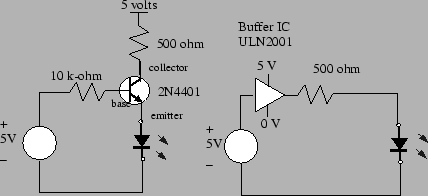Another type of LED driver is shown in the right hand circuit of figure 19. This circuit uses an integrated circuit known as a Darlington current driver (ULN2001) to drive the LED. It is, essentially, the same as the left hand circuit with the exception that we've replaced the single npn transistor by an integrated circuit that can provide a much much larger current than could have been sourced by the single transistor. The other difference is that the ULN2001 does not act as an inverter to the LED is turned on when we set the input line high.Next: Learning Module Exercise Up: Analog and Digital Interfacing Previous: Mechanical Switches
Bill Goodwine 2002-09-29